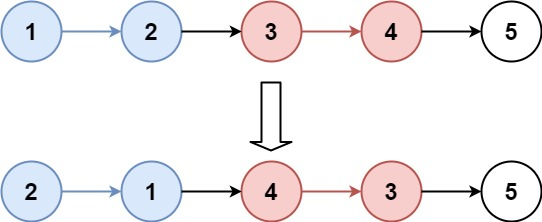## Algorithm

Problem Name: 25. Reverse Nodes in k-Group

Given the `head` of a linked list, reverse the nodes of the list `k` at a time, and return the modified list.

`k` is a positive integer and is less than or equal to the length of the linked list. If the number of nodes is not a multiple of `k` then left-out nodes, in the end, should remain as it is.

You may not alter the values in the list's nodes, only nodes themselves may be changed.

Example 1:```Input: head = [1,2,3,4,5], k = 2
Output: [2,1,4,3,5]
```

Example 2:```Input: head = [1,2,3,4,5], k = 3
Output: [3,2,1,4,5]
```

Constraints:

• The number of nodes in the list is `n`.
• `1 <= k <= n <= 5000`
• `0 <= Node.val <= 1000`

## Code Examples

### #1 Code Example with C Programming

```Code - C Programming```

``````
struct ListNode* reverseKGroup(struct ListNode* head, int k) {
struct ListNode *node, *next;
struct ListNode **stack;
struct ListNode dummy = { 0 }, *p;
int i;

if (k <= 1) return head;

stack = malloc(k * sizeof(struct ListNode *));
//assert(stack);

#define PUSH(NODE) do { stack[i++] = NODE; } while (0)
#define POP(NODE) do { NODE = stack[--i]; } while (0)

p = &dummy;

while (node) {
i = 0;
while (i < k && node) {
PUSH(node);
node = node->next;
}
next = node;

if (i < k) {
free(stack);
return dummy.next;
}

while (i > 0) {
POP(node);
p->next = node;
p = node;
}
p->next = node = next;
}

free(stack);
return dummy.next;
}
``````
Copy The Code &

Input

cmd
head = [1,2,3,4,5], k = 2

Output

cmd
[2,1,4,3,5]

### #2 Code Example with C++ Programming

```Code - C++ Programming```

``````
class Solution {
public:
ListNode* reverseKGroup(ListNode* head, int k) {
ListNode* pre = new ListNode(0);
ListNode* res = pre;
while(fast){
int i = k - 1;
while(fast && i--) fast = fast->next;
if(fast){
next = fast->next;
reverse(pre, slow, fast, next);
pre = slow;
slow = next;
fast = next;
}
}
return res->next;
}

void reverse(ListNode* left, ListNode* start, ListNode* end, ListNode* right){
ListNode* pre(left), *cur(start), *next;
ListNode* tmp = start;
while(cur != right){
next = cur->next;
cur->next = pre;
pre = cur;
cur = next;
}
left->next = pre;
tmp->next = right;
}
};
``````
Copy The Code &

Input

cmd
head = [1,2,3,4,5], k = 3

Output

cmd
[3,2,1,4,5]

### #3 Code Example with Java Programming

```Code - Java Programming```

``````
class Solution {
public ListNode reverseKGroup(ListNode head, int k) {
ListNode prev = null;
while (curr != null) {
ListNode start = curr;
int idx = 1;
for (idx = 1; idx < k && curr.next != null; idx++) {
curr = curr.next;
}
if (idx != k) {
curr = null;
continue;
}
ListNode next = curr.next;
curr.next = null;
ListNode reversedNode = reverse(start);
}
if (prev != null) {
prev.next = reversedNode;
}
prev = start;
start.next = next;
curr = next;
}
}

private ListNode reverse(ListNode node) {
ListNode curr = node;
ListNode prev = null;
while (curr != null) {
ListNode next = curr.next;
curr.next = prev;
prev = curr;
curr = next;
}
return prev;
}
}

``````
Copy The Code &

Input

cmd
head = [1,2,3,4,5], k = 2

Output

cmd
[2,1,4,3,5]

### #4 Code Example with Javascript Programming

```Code - Javascript Programming```

``````
const reverseKGroup = function(head, k) {
let n = 0
for (let i = head; i != null; n++, i = i.next);
let dmy = new ListNode(0)
for (let prev = dmy, tail = head; n >= k; n -= k) {
for (let i = 1; i < k; i++) {
let next = tail.next.next
tail.next.next = prev.next
prev.next = tail.next
tail.next = next
}

prev = tail
tail = tail.next
}
return dmy.next
}
``````
Copy The Code &

Input

cmd
head = [1,2,3,4,5], k = 3

Output

cmd
[3,2,1,4,5]

### #5 Code Example with Python Programming

```Code - Python Programming```

``````
class Solution:
dummy = last = ListNode(0)
while cur:
first, cnt = cur, 1
while cnt < k and cur:
cur, cnt = cur.next, cnt + 1
if cnt == k and cur:
cur, prev = first, None
for _ in range(k):
prev, cur.next, cur = cur, prev, cur.next
last.next, last = prev, first
else:
last.next = first
return dummy.next
``````
Copy The Code &

Input

cmd
head = [1,2,3,4,5], k = 2

Output

cmd
[2,1,4,3,5]

### #6 Code Example with C# Programming

```Code - C# Programming```

``````
namespace LeetCode
{
public class _025_ReverseNodesInKGroup
{
public ListNode ReverseKGroup(ListNode head, int k)
{
if (k <= 1) { return head; }

ListNode p = dummyHead, q, r;
int i = 0;

while (p.next != null)
{
q = p.next;
for (i = 0; i < k; i++)
{
if (q == null) { return dummyHead.next; }
q = q.next;
}

q = p.next;
for (i = 1; i < k; i++)
{
r = q.next;
q.next = r.next;
r.next = p.next;
p.next = r;
}

p = q;
}

}
}
}
``````
Copy The Code &

Input

cmd
head = [1,2,3,4,5], k = 3

Output

cmd
[3,2,1,4,5]